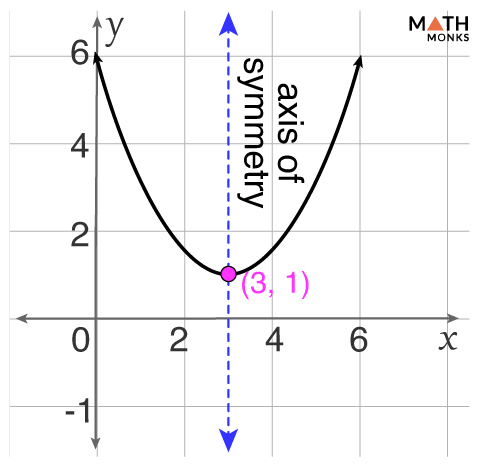# Axis of Symmetry

The axis of symmetry is an imaginary line that makes the shape symmetrical about it. In other words, it divides the shape into two halves such that each half is a mirror image of the other. If we fold and unfold an object along the axis of symmetry, the two sides are identical.

Different geometric shapes have different lines of symmetry.

• A square has 4 lines of symmetry
• A rectangle has 2 lines of symmetry
• A circle has infinite lines of symmetry
• A parallelogram has no line of symmetry
• A regular polygon with ‘n’ sides has ‘n’ axes of symmetry

## In Parabola

The axis of symmetry is best studied in a parabola, while graphing a quadratic function. Thus, the axis of symmetry of a parabola is the line about which a parabola is symmetric. It always passes through its vertex. The x -coordinate of the vertex is the equation of the parabola’s axis of symmetry.

The axis of symmetry determines the form of the parabola. It can be either horizontal or vertical, facing left or right.

• If the axis of symmetry is vertical, the parabola is vertical that opens either up or down)
• If the axis of symmetry is horizontal, the parabola is horizontal that opens left or right.

Now, we will discuss how to find the axis of symmetry of parabola from its standard and vertex forms of equations.

### Formulas

#### From Standard Form of the Equation

The standard form of a quadratic equation is y = ax2+ bx + c,

The equation of axis of symmetry is,

• x = – b/2a, here a = coefficient of x2, b = coefficient of x, c = constant

#### From Vertex Form of the Equation

The vertex form of a quadratic equation is y = a(x – h)2 + k,

Equation of axis of symmetry is,

• x = h, here (h, k) = vertex of the parabola.

We obtain the vertex of the function (x, y) by substituting the value of x in the standard form of the equation and get the value of y.

Let us solve some examples involving the above formulas and concepts.

## Solved ExamplesFind the axis of symmetry and vertex of the graph of the quadratic function y = x2 +2x +11. Draw the graph.

Solution:As we know,
The equation is in the standard form,
∴ The axis of symmetry is,
x = -b/2a, here b = 2, a = 1
= -2/2 × 1
= -1
Substituting the value of x in the equation y = x2 +2x +11
∴ y = (-1)2 +2(-1) +11
= 1 – 2 + 11
= 10
So the vertex is (-1, 10)Determine the axis of symmetry of the graph of ${y=\dfrac{2}{3}\left( x-3\right) ^{2}+1}$. Draw the graph.

Solution:As we know,
The equation is in the vertex form,
${y=\dfrac{2}{3}\left( x-3\right) ^{2}+1}$,
∴ axis of symmetry is x = h, here (h, k) = (3, 1)
∴ x = 3
The graph is drawn in the figure alongside.